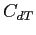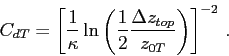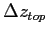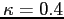Next: CdB: Up: Physical/Computational parameters Previous: z0B:   Contents

### CdT:If the roughness at the lid is zero (i.e. z0T=0) then the drag coefficient at the lid is given by this constant, otherwise the drag coefficient is calculated with the rough wall relationship. Use of no-slip boundary conditions for the top cell is specified by setting CdT=-1.whereis the thickness of the top cell (in meters) andis the von Karman constant.

2014-08-06